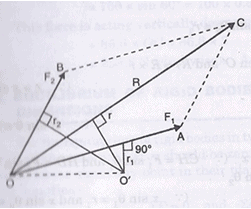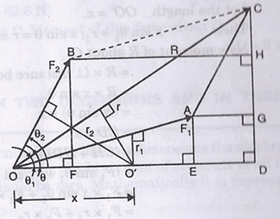Courses

# Principle of Moments Mechanical Engineering Notes | EduRev

## Mechanical Engineering : Principle of Moments Mechanical Engineering Notes | EduRev

The document Principle of Moments Mechanical Engineering Notes | EduRev is a part of the Mechanical Engineering Course Engineering Mechanics - Notes, Videos, MCQs & PPTs.
All you need of Mechanical Engineering at this link: Mechanical Engineering

Principle of Moments or Varignon’s Theorem

Varignon’s Theorem states that the moment of a force about any point is equal to the algebraic sum of the moments of its components about that point.

Principal of moments states that the moment of the resultant of a number of forces about any point is equal to the algebraic sum of the moments of all the forces of the system about the same point.

Proof of Varignon’s TheoremFig. 2.34 (a)Fig.2.34 (b)

Fig2.34 (a) shows two forces Fj and F2 acting at point O. These forces are represented in magnitude and direction by OA and OB. Their resultant R is represented in magnitude and direction by OC which is the diagonal of parallelogram OACB. Let O’ is the point in the plane about which moments of F1, F2and Rare to be determined. From point O’, draw perpendiculars on OA, OC and OB.

Let r1= Perpendicular distance between F1 and O’.

r2= Perpendicular distance between R and O’.

r3= Perpendicular distance between F2 and O’.

Then according to Varignon’s principle;

Moment of R about O’ must be equal to algebraic sum of moments of F1 and F2about O’.

R * r = F1 * r1 + F2 * r2

Now refer to Fig. 2.34 (b). Join OO’ and produce it to D. From points C, A and B draw perpendiculars on OD meeting at D, E and F respectively. From A and B also draw perpendiculars on CD meeting the line CD at G and H respectively.

Let  θ1 = Angle made by F; with OD,

θ = Angle made by R with OD, and
θ2 = Angle made by F2with OD.

In Fig. 2.34 (b), OA = BC and also OA parallel to BC, hence the projection of OA and BC on the same vertical line CD will be equal i.e., GD = CH as GD is the projection of OA on CD and CH is the projection of BC on CD.

Then from Fig. 2.34 (b), we have

P1 sin θ1 = AE = GD = CH
F1 cos θ1 = OE

F2 sin θ1 = BF = HD
F2 cos θ2 = OF = ED

(OB = AC and also OB || AC. Hence projections of OB and AC on the same horizontal line OD will be equal i.e., OF = ED)

sin θ =CD
cos θ =OD

Let the length OO’ = x.

Then    x sin θ1 = r, x sin θ = r and x sin θ2 = r2

= R * (distance between O’ and R) = *

= R * sin θ                          (r = sin θ)

=(R sin θ) *

= CD x                              (Rsin θ = CD)

= (CH +HD)* x

= (F1 sin θ1 + F2 sin θ2) * x            (  CH = F1 sin θ1 and HD = F2 sin θ2)

= F1* sin θ1 + F2* sin θ2

= F1 *r+ F*r2         (  x sin θ= rand x sin θ= r2)

Hence moment of about any point in the algebraic sum of moments of its components i.e., F1and F2)about the same point. Hence Varignon’s principle is proved.

The principle of moments (or Varignon’s principle) is not restricted to only two concurrent forces but is also applicable to any coplanar force system, i.e., concurrent or non-concurrent or parallel force system.

Offer running on EduRev: Apply code STAYHOME200 to get INR 200 off on our premium plan EduRev Infinity!

,

,

,

,

,

,

,

,

,

,

,

,

,

,

,

,

,

,

,

,

,

;